Refer to our Texas Go Math Grade 6 Answer Key Pdf to score good marks in the exams. Test yourself by practicing the problems from Texas Go Math Grade 6 Lesson 7.2 Answer Key Rates.

Reflect

Question 1.
Analyze Relationships Describe another method to compare the costs.
The total cost can be divided by the total number of ounces. The result of this division will be the cost per ounce or cost of 1 ounce The greater this number, the more expensive the juice is.

Question 2.
Analyze Relationships In all of these problems, how is the unit rate related to the rate given in the original problem?
Unit rate multiplied by the number of ounces equals to the given rate.

Question 3.
There are 156 players on 13 teams. How many players are on each team? ________ players per team
In order to find how many players are in each team,
we have divide 156 by 13.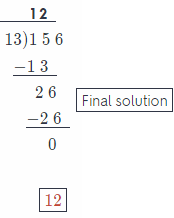12 players in each term

Question 4.
A package of 36 photographs costs $18. What is the cost per photograph?$ _______ per photograph
To find the unit rate, divide the numerator and denominator by 2:The cost per photograph is $2. Reflect Question 5. What If? Suppose each group has 12 campers and 3 canoes. Find the unit rate of campers to canoes. Answer: To find the unit rate, divide the numerator by 3: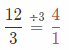= $$\frac{4}{1}$$ Question 6. Petra jogs 3 miles in 27 minutes. At this rate, how long would it take her to jog 5 miles? Answer: To find the unit rate, divide the numerator and denominator by 3: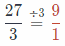Multiply the numerator and denominator by 5 to find the solution: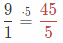It would take 45 minutes. Question 7. When Jerry drives 100 miles on the highway, his car uses 4 gallons of gasoline. How much gasoline would his car use if he drives 275 miles on the highway? Answer: To find the unit rate, divide the numerator and denominator by 100:Multiply the numerator and denominator by 5 to find the solution: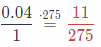It would use 11 gallons of gasoline. Texas Go Math Grade 6 Lesson 7.2 Guided Practice Answer Key The sizes and prices of three brands of laundry detergent are shown in the table. Use the table for 1 and 2.Question 1. What is the unit price for each detergent? Brand A:$ ________________ per ounce
Brand B: $________________ per ounce Brand C:$ ________________ per ounce
A: To find the unit rate, divide the numerator and denominator by 32: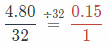$0.15 per ounce. B: To find the unit rate, divide the numerator and denominator by 48: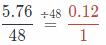$ 0.12 per ounce.

C: To find the unit rate, divide the numerator and denominator by 48:$0.14 per ounce. Question 2. Which detergent is the best buy? ________________ Answer: Brand B. (lowest price per ounce) Mason’s favorite brand of peanut butter is available in two sizes. Each size and its price are shown in the table. Use the table for 3 and 4.Question 3 What is the unit rate for each size of peanut butter? Regular:$ _________________ per ounce
Family size: $_________________ per ounce Answer: Form the rate fraction by using the given data, therefore: Rate = $$\frac{\text { Cost }}{\text { Quantity }}$$ Substitute values for Regular: Rate = $$\frac{3.36}{16}$$ = 0.21 This implies that the unit rate for regular size peanut butter is$0.21 per ounce.

Form the rate fraction by using the given data, therefore:
Rate = $$\frac{\text { Cost }}{\text { Quantity }}$$
Substitute values for Regular:
Rate = $$\frac{7.6}{40}$$ = 0.19
This implies that the unit rate for regular size peanut butter is $0.19 per ounce. Question 4. Which size is the better buy? Answer: 0.21 > 0.19 impLies that the family size is a better buy because it costs lesser per ounce than the regular size one. Find the unit rate. (Example 1) Question 5. Lisa walked 48 blocks in 3 hours. _____________ blocks per hour Answer: Find the unit rate. How many blocks Lisa passes in an hour Divide to find the unit rate $$\frac{48 \text { blocks }}{3 \text { hours }}=\frac{16 \text { blocks }}{1 \text { hours }}$$ Liza passes 16 blocks in an hour Final solution = 16 Question 6. Gordon types 1,800 words in 25 minutes. _____________ words per minute Answer: Find the unit rate. How many words he types per minute Divide to find the unit rate $$\frac{1800 \text { words }}{25 \text { minutes }}=\frac{72 \text { words }}{1 \text { minute }}$$ Gordon types 72 words per minute. Final solution = 72 Solve. Question 7. A particular frozen yogurt has 75 calories in 2 ounces. How many calories are in 8 ounces of the yogurt? Answer: Find the unit rate. How much calories are in one ounce Divide to find the unit rate $$\frac{75 \text { calories }}{2 \text { ounces }}=\frac{37.5 \text { calories }}{1 \text { ounce }}$$ Yogurt has 37.5 calories in 1 ounce 37.5 calories × 8 ounces = 300 calories In yogurt there are 300 calories Final Solution = 300 Question 8. The cost of 10 oranges is$1.00. What is the cost of 5 dozen oranges?
Form the rate fraction by using the given data. therefore:
Rate = $$\frac{\text { Calories }}{\text { Ounces }}$$
Substitute values:
Rate = $$\frac{1}{10}$$ = 0.1
Unit rate is equal to $0.1 per orange. 5 dozens of oranges are 5 × 12 = 60. Therefore Go oranges will cost 60 ×$0.1 = $6. 5 dozens of oranges will cost$6.

Question 9.
On Tuesday, Donovan earned $11 for 2 hours of babysitting. On Saturday, he babysat for the same family and earned$38.50. How many hours did he babysit on Saturday?
To find the unit rate, divide the numerator and denominator by 2: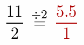For every hour lie earns 5.5$. Multiply the numerator and denominator by 7 to find the solution: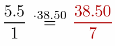He babysat 7 hours on Saturday. Essential Question Check-In Question 10. How can you use a rate to compare the costs of two boxes of cereal that are different sizes? Answer: Divide the cost of the cereal box with the total amount of cereal in the box for both boxes. This will give the cost per amount. The larger this number, the more expensive the cereal box is with respect to its content. Question 11. Abby can buy an 8-pound bag of dog food for$7.40 or a 4-pound bag of the same dog food for $5.38. Which is the better buy? Answer: Evaluate the unit rate of each bag. Therefore the 8 pounds bags costs $$\frac{\ 7.40}{8}$$ =$ 0.925 per pound, while the 4 pounds bags costs = $$\frac{\ 5.38}{4}$$ = $1 .345 per pound. Since 1.315 > 0.925. the 8 pounds bag is a better buy because it costs lesser per pound. 8 pound bag is a better buy. Question 12. A bakery offers a sale price of$3.50 for 4 muffins. What is the price per dozen?
To find the unit rate, divide the numerator and denominator by 4: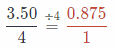The cost per muffin is = $0.875 . Multiply the numerator and denominator by 12 to find the solution: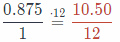The cost per dozen is$ 10.50

Taryn and Alastair both mow lawns. Each charges a flat fee to mow a lawn. The table shows the number of lawns mowed in the past week, the time spent mowing lawns, and the money earned.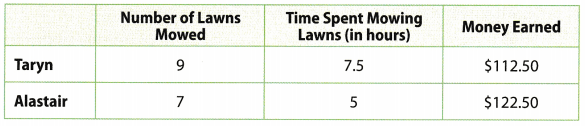Question 13.
How much does Taryn charge to mow a lawn?
Find the unit rate. How much does Taryn charge for lawn?

Divide to find the unit rate
$$\frac{112.5 \text { dollars }}{9 \text { lawns }}=\frac{12.5 \text { dollars }}{1 \text { lawn }}$$

Tarny charges 12.5 dollars for lawn
Final solution = 12.5

Question 14.
How much does Alastair charge to mow a lawn?
Find the unit rate. How much does Alastair charge for the lawn?

Divide to find the unit rate
$$\frac{122.5 \text { dollars }}{7 \text { lawns }}=\frac{17.5 \text { dollars }}{1 \text { lawn }}$$

Alastair charges 17.5 dollars for lawn
final solution = 17.5

Question 15.
Who earns more per hour, Taryn or Alastair?
Alastair earns more per hour. It can be seen that he has earned $122.5 >$ 112.5 after moving for 5 hours then Taryn earned after working for 7.5 hours.

Question 16.
What If? If Taryn and Alastair want to earn an additional $735 each, how many additional hours will each spend mowing lawns? Explain. Answer: Alastair earns 17.5 per hour. Multiply the denominator and numerator by 42 to find the solution:$: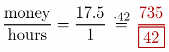Taryn earns $12.5 per hour. Multiply the denominator 58.8 to find the solution:$:Alastair: 42 hours
Taryn: 58.8 hours

Question 17.
Multistep Tomas makes balloon sculptures at a circus. In 180 minutes, he uses 252 balloons to make 36 identical balloon sculptures.
a. How many minutes does it take to make 1 balloon sculpture?
To find the unit rate, divide the numerator and denominator by 36: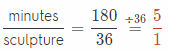It takes 5 minutes.

b. How many balloons are used in one balloon sculpture?
To find the unit rate, divide the numerator and denominator by 36:7 ballons.

c. What is Tomas’s unit rate for balloons used per minute?
To find the unit rate, divide the numerator and denominator by 180: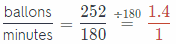Question 18.
Quan and Krystal earned the same number of points playing the same video game. Quan played for 45 minutes and Krystal played for 30 minutes. Whose rate of points earned per minute was higher? Explain.
Krystal’s rate of points earned per minute is higher. Number of points divided by 30 minutes will give higher rate then divided by 45 minutes.

For example, let number of points equals to 90.
Krystal’s rate of points:
$$\frac{90}{30}=\frac{3}{1}$$
Quan’s rate of points:
$$\frac{90}{45}=\frac{2}{1}$$

Mrs. Jacobsen is a music teacher. She wants to order toy instruments online to give as prizes to her students. The table below shows the prices for various order sizes.Question 19.
What is the highest unit price per kazoo?
25 items:
To find the unit rate, divide the numerator and denominator by 25:The cost per item is $0.4 50 items: To find the unit rate, divide the numerator and denominator by 50: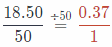The cost per item is$ 0.37

80 items:
To find the unit rate, divide the numerator and denominator by 80:The cost per item is $0.34 The highest price is$ 0.4

Question 20.
Persevere in Problem Solving If Mrs. Jacobsen wants to buy the item with the lowest unit price, what item should she order and how many of that item should she order?
She Should order 80 kazoos.

H.O.T. Focus On Higher Order Thinking

Question 21.
Draw Conclusions There are 2.54 centimeters in 1 inch. How many centimeters are there in 1 foot? in 1 yard? Explain your reasoning.
There are 254 centimeters in 1 inch, and there are 12 inches in 1 foot, so there are 2.54 × 12 = 30.48 centimeters in 1 foot.

There are 30.48 centimeters in 1 foot and there are 3 feet in a yard, so there are 3 × 30.48 = 91.44 centimeters in 1 yard.

Question 22.
Critique Reasoning A 2 pound box of spaghetti costs $2.50. Philip says that the unit cost is $$\frac{2}{2.50}$$ =$0.80 per pound. Explain his error.
He has divided the total pounds with the total price giving pounds of spaghetti per dollar instead of unit cost. The correct rate is $$\frac{2.5}{2}$$ = $1 .25 per pound. He divided the total pounds with the total price. Question 23. Look for a Pattern A grocery store sells three different quantities of sugar. A 1-pound bag costs$1.10, a 2-pound bag costs $1.98, and a 3-pound bag costs$2.85. Describe how the unit cost changes as the quantity of sugar increases.
Cost of 1 pound in the 1 pound bag $$\frac{\ 1.10}{1}$$ = $1.10 Cost of 1 pound in the 2 pounds bag $$\frac{\ 1.98}{2}$$ =$ 0.99
Cost of 1 pound in the 3 pounds bag $$\frac{\ 2.85}{3}$$ = \$ 0.95